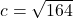## If it takes Ohenhen 10km to pass to Emma and it takes Emma 8km to pass to Omusi and considering Ohenhen & Omusi are at perpendicular end

Question

If it takes Ohenhen 10km to pass to Emma and it takes Emma 8km to pass to Omusi and considering Ohenhen & Omusi are at perpendicular ends from Emma. What’s the distance between Ohenhen and Omusi?​

in progress 0
2 months 2021-08-01T11:22:44+00:00 1 Answers 4 views 0

12.81 km

Step-by-step explanation:

Pythagoras formula for right-angled triangles.

this scenario just says that Emma is the corner point of that triangle with an angle of 90 degrees.

the distance between Ohenhen and Omusi is the baseline (Hypotenuse c) of that triangle. and the distances to Emma are the sides a and b.

so the formula is

c² = a² + b² = 10² + 8² = 100 + 64 = 164c ≈ 12.81 km (rounded to the nearest hundredth).